Articles

# Improved precision of hydraulic conductance measurements using a Cochard rotor in two different centrifuges

## Abstract

An improved way ofcalculating hydraulic conductance (K) in a Cochard cavitron is described. UsuallyK is determined by measuring how fast water levels equilibrate between two reservoirs while a stem spins in a centrifuge. A regression of log meniscus position versus time was used to calculate K and this regression method was compared to the old technique that takes the average of discrete values. Results of a hybrid Populus 84K shows that the relative error of the new approach is significantly lower than the old technique by 4~5 times. The new computational method results in a relative error less than 0.5% or 0.3% from 8 or 12 points of measurement, respectively. The improved precision of K measurement also requires accurate assessment of stem temperature because temperature changes K by 2.4% oC-1. A computational algorithm for estimating stem temperature stability in a cavitron rotor was derived. This algorithm provides information on how long it takes stem temperature to be known to within an error of ±0.1oC.

## Introduction

The cavitron technique has been used for many years to measure vulnerability curves (VCs) of woody stems (Cochard 2002, Cochard, Damour et al. 2005). VCs have been widely viewed as a good measure of the drought resistance of woody plants (Cochard et al. 2013). Increasing drought will induce cavitation of water held in stem conduits (vessels or tracheids). A cavitation event occurs when water columns break under tension (=minus the pressure of water in xylem). A cavitated vessel first fills with water vapor then eventually fills with air-bubbles at atmospheric pressure because of Henry’s law of gas solubility at water/air interfaces. The time required for equilibrium of air-pressure depends on the rate of air penetration into the recently-cavitated vessel lumen via diffusion in the liquid phase (Fick’s Law).

We have been conducting experiments that address the tempo of air bubble formation in recently cavitated vessels. The equilibrium for air bubble formation is defined by Henry’s law, from which we can predict that eventually the air pressure inside a cavitated vessel must equal ambient atmospheric pressure. While doing initial experiments we realized we needed to improve the precision of hydraulic conductance (K) measurement in stems spinning in a Cochard cavitron in order to study how long it takes air bubbles to form. Current measurements are reproducible to about 2% (see for example Cai et al 2014) over short periods of time (minutes). Over longer periods of time (1-2 h) K can also be influenced by the change of temperature in the centrifuge while the rotor is spinning due to non-stable temperature control in some centrifuges. If a spinning stem experiences a temperature change of ± 1 oC then this will cause a change in measured K of ±2.4% because of the affect of temperature on the viscosity of water near room temperature.

## Materials and Methods

### Theory

Hydraulic conductance, K, can be measured in a cavitron at both high negative pressure and low (sub-atmospheric) pressure. A multi-point measurement of K can be completed in 20 to 60 seconds and hence we can assume K is constant during the measurement. In the traditional method, K is measured several times over small time and volume increments by observing two menisci: one that is stationary and the other that moves towards the stationary meniscus a distance x away relative to the stationary meniscus. During the measurement the meniscus moves a distance Δx in a cuvette in a time interval Δt and the flow rate is evaluated from F = rAwΔx/Δt (kg s-1) where r is the density of water, and Aw is the cross sectional area of water in the cuvette. At the same time the pressure drop causing flow is computed from ΔP = 0.5ρω2(2R-2) where is the value of x at the midpoint of Δx. In this equation ω is the angular velocity of the rotor spinning, and R = the maximum radius from the axis of rotation to the lower stationary meniscus. In Cochard’s original equations a lower case ‘r’ is used in place of x. The value of K is calculated from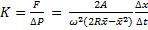(1)

The problem with Eq. (1) is that the time and distance intervals are rather small and hence the standard deviation on repeated measurements of Δx/Δt is rather large (around 10%).

One of us (YW) noticed during experiments that x (the distance of the moving meniscus from stationary meniscus) declines exponentially with time towards 0 at constant ω, i.e., a plot of ln(x) declines linearly with t. This behavior follows from Eq. (1) written in differential format (dx/dt = Δx/Δt) when we neglect x2, which is small compared to 2Rx (typically x < 3 versus 2R = 254 mm). So we tried measuring the slope, m, of a plot of ln(x) versus t and computed K from the solution of differential Eq. (1) ignoring x2. The solution of Eq(1) ignorning x2 is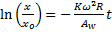(2A)

Hence a plot of the ln(x/xo) versus t will yield a slope, m, from which we can compute K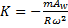(2B)

The linear regression provides only one value for the slope, but a standard regression analysis can be used to compute the standard error of the slope, m. Preliminary experiments demonstrated to us that the computation of K from Equation (2) had a much smaller SE than from repeated measurements of Eq. (1). So we started looking for the exact solution to differential equation resulting from Eq. (1):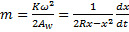(3)

The solution provided by (RB) is: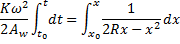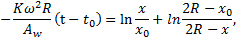(4)

where t0 is equated to zero, i.e., the time when we begin to record the movement of the upper meniscus (at x0), and t is the time when we record the passage of the meniscus at position x0. The second natural log term in Eq.(4) contributes 1 to 2% to the slope depending on the range of x. Hence our initial use of just ln(xo/x) = constant –ln(x) was approximately correct but the exact solution is preferable. Theoretically t should be proportional to

y =, with a slope, m =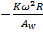. From the recorded x vs t, we compute y vs t and from the regression we got the slope, m, to calculate K as shown in Eq. (2B) above.

### Experiments for computation of K

Test experiments were conducted on clonal hybrid Populus 84K shoots (Populus alba × Populus glandulosa). Branches were cut from the trees growing on the campus of Northwest A&F University. Segments of 0.274 m long and 6-7 mm in basal diameter were cut from harvested branches (> 0.5 m long) under water, and were fully flushed with 10 mM KCl under 200 kPa pressure for 30 min.

A Cochard cavitron was used to test our improved method, in which we used a 20X or 40X microscope to observe the water level changes. The difference between two holes on the two reservoirs was 6.5 mm or 3.8 mm depending on the microscope used. Some measurements were performed on a cavitron rotor in a Beckmann Coulter model Allegra X-22R centrifuge and the temperature-dependent experiments were done in a Xiang Yi model H2100R centrifuge because it had better temperature regulation.

We measured vulnerability curves to see whether hydraulic conductance obtained by two methods (regression and traditional) were the same at different tensions. We collected typically 11 points of x versus t and obtained the means and SE of the slope by the regression method and the mean and SE by the traditional method using 10 values of Δx and Δt and equation (1) to compute K and then computed the traditional mean and SE.

Since the two methods manipulate the same data set, it is essential to recognize that the methods being compared are differentiated by a purely computational difference except in one way. In the old method Δx and Δt (Eq. 1) are usually determined 6 to 11 times by refilling the cuvette 2 or 3 times during the sequence of measurements and applying Eq. (1) 6 to 11 times. In this study the cuvette is filled only once and 8 to 11 measurements of Δx and Δt are made, which provides data sets that can be evaluated by use of Eq. (1) 8 to 11 times and this is compared to a regression using Eq. (4). Since the time required for the meniscus to move a fixed distance, Δx, increases as the experiment progresses the value of Δt also increases, so successive measurements of K using Eq. (1) become more accurate as the measurements progress. This should make the ratio of (standard error)/(mean K) smaller hence giving a more accurate mean K. Despite this trend we will demonstrate that the ratio decreases faster by the regression-computational method.

### Temperature tests: an algorithm to estimate Tstem

Enhanced precision of measurement of K is of little value if the stem temperature, Tstem, is unknown and variable because K is proportional of 1/η where η is the viscosity of water. The value of 1/η changes about 2.4% oC-1 near 20 oC. The temperature depedence of 1/η ranges from 3.3 to 1.95 % oC-1 for temperatures from 0 to 40 oC, respectively. Hence Tstem must be known or controlled to within ±0.1 oC to achieve ±0.24% accuracy in the computation of percent loss of conductance (PLC) which needs to be determined by repeated measurements of K at the same Tstem but differing tensions during the construction of a vulnerability curve.

The approach we took was to make use of the fact that a stem is a defacto thermometer when repeated measurements of K are made at low tension, i.e., when only temperature affects K. Most centrifuges have refrigeration (or a heat pump that can both heat and cool) and a thermostatic way to set and control temperature. But thermostatic control of temperature is never completely precise. In order to assess the impact of fluctuations of temperature inside a centrifuge on Tstem, we programmed large changes in temperature and monitored changes in K vs time while measuring the air temperature inside the centrifuge with a temperature sensor near the rotor. Many types of temperature sensors are capable of ±0.1 oC resolution after calibration; these include thermistors, thermocouples and LM335 chips; we used the latter. But air temperature will not reflect the likely temperature of the spinning stem.  Hence we tried to devise a computational algorithm to compute Tstem adequately to predict the observed changes in K. Then this algorithm was used to assess the ability of different centrifuges to control Tstem.

We tested two kinds of computational algorithms: The first was a running mean of the air-temperature in the centrifuge. We monitored air-temperature every 5 seconds, and we computed the running mean air temperature for various length of time. The second algorithm was a first-order rate reaction equation where the change in stem temperature at any time interval is given by

ΔTstem= αΔt(Tair,i-Tstem,i),

where α = the ‘heat transfer’ rate constant and Δt is the time step. Hence if we know Tstem at time t=0 (Tstem,0) then the stem temperature at later time (Tstem,t) after n measuring intervals is given by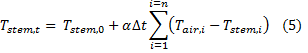In the first algorithm nothing is said about the initial stem temperature (Tstem,0) but it is assumed that if we hold air temperature constant long enough then a running mean of some time-period can be found to predict Tstem within ±0.1 oC and this running mean length can be determined experimentally. In the second algorithm we do not need to know Tstem,0 but if we hold the temperature constant long enough then the sum in Eq.(6) will make the value of Tstem,t converge on the real Tstem within ±0.1 oC. Experiments were done to see if the first or second algorithm or a combination of the two algorithms can be used to predict Tstem when Tair is dynamically changing.

## Results and Discussion

Figure 1 shows the values of K versus tension in Populus clone 84K which is typical of a vulnerability curve measured in July because in July the stem contains current-year and previous-year vessels which differ in ‘P50’ because of frost fatigue (unpublished results). Similar data have been obtained on >10 branches.  Data for K were computed by both methods: regression (Eq. 4) and traditional (mean of discrete measurement in Eq. (1)). We concluded that hydraulic conductance acquired by the two methods were the same but the regression method was more precise. The residuals were randomly distributed indicating that the regression approach can give unbiased values that were independent of hydraulic conductance. The difference between K measured by the regression versus traditional methods was less than 0.3% but the precision of the regression method was superior. The error in (SE/Mean) of the regression method was smaller than the traditional method by a factor of 4~5 times as shown in Fig. 1C, which means that the regression method was 4 to 5 times more ‘precise’.

Figure 2 shows four examples of the regression fitting of y versus t,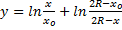, including linear fitting and residual distribution of two examples at the beginning of the curve with tension at 0.088 and 0.127 MPa and two examples at the ending of the curve with tension at 2.207 and 2.387 MPa respectively. Values of R2 for the four fittings were all > 0.999 and were typically >0.9999 except at the lowest K-values, and the residuals were random in Figure 2B and a little shifted in Figure 2D. Residuals in Figure 2D were not quite random because of the very low hydraulic conductance at the end of the vulnerability curve and because new embolism may have been occurring during the progress.Figure 1. This shows values of K* obtained from a regression (Eq. 4) method versus the value of K obtained by the traditional method (Eq. 1). Panel A: This shows a plot of K (closed circle) and K* during the measurement of a vulnerability curve. Panel B: This shows the linear regression of K and K*, the slope was nearly one (SE = 1.408E-3 and p = 1.8E-47); the intercept was not significantly different from zero (SE =1.5E-7 and p = 0.9215). Panel C: This shows the comparison of relative error (SE/Mean) of the regression versus traditional method. Panel D: This shows the residual of the linear regression in panel B.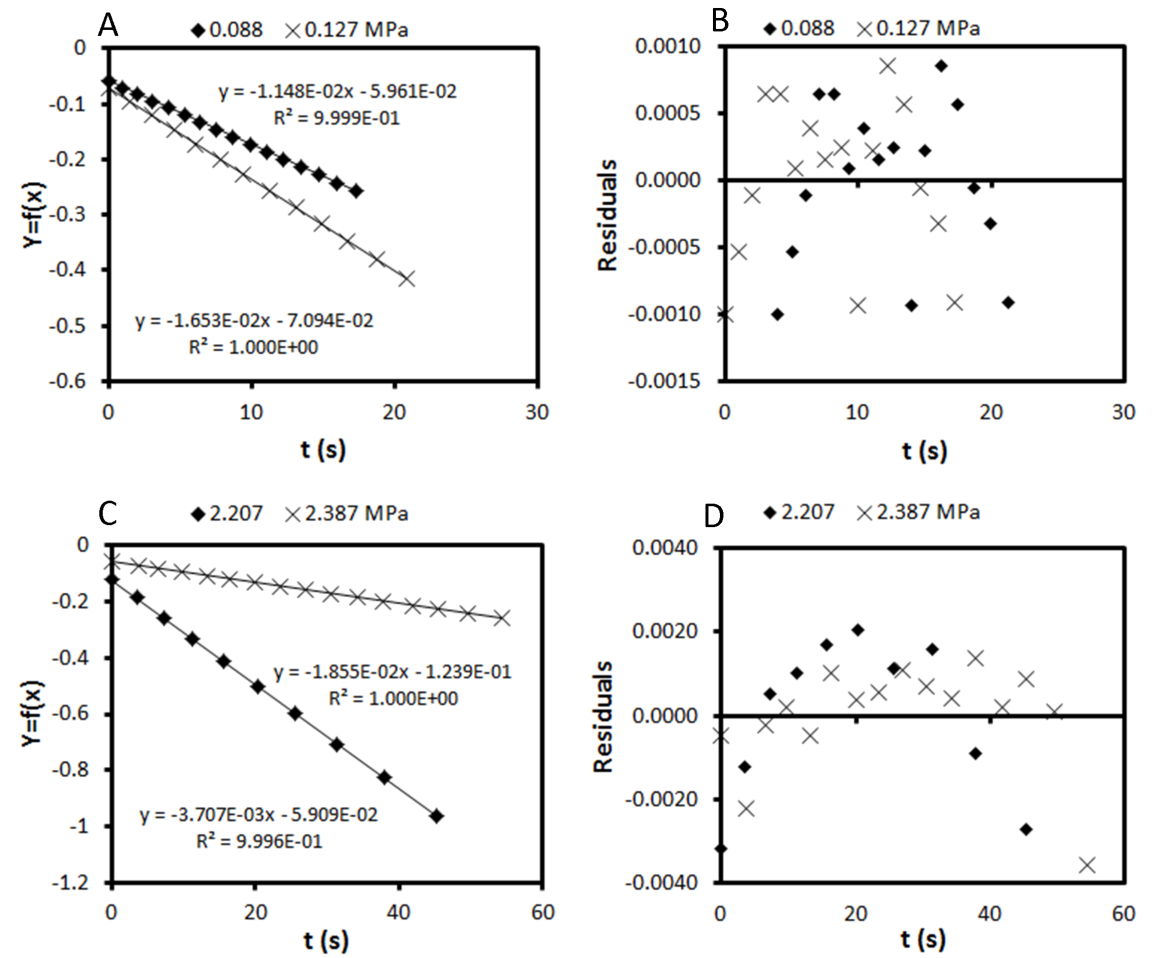Figure 2. This shows examples of linear regressions used to predict hydraulic conductance. Panel A: two examples at tension 0.088 and 0.127 MPa at the beginning of a vulnerability curve, and panel B: the residuals of the linear regressions in panel A. Panel C: two examples at 2.207 and 2.387 MPa at the end of a vulnerability curve, and panel D: the residuals of the linear regression in panel C. In panel A and C,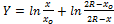where x0 is the distance between two menisci at the beginning of the measurement, x is the distance of water levels at time t, R is the distance from rotational axis to the lowest water level in the cuvette with the lowest hole.

Figure 3 shows how the error changed with the number of points used in a regression. To evaluate the impact of the number of points on SE/meanK (SE/), we choose 5 to 11 points from every original curve in the vulnerability curve to compare how SE/ changed with N. At a given number of points, N, regression means and errors were calculated by least square, and SE/ of traditional method were computed by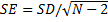(N was the number of recorded times or distances, so actually the total number of discrete hydraulic conductance used for average approach was N-1). Furthermore we compared the average of the first, second and third part of the SE/means, which separately referred to the begining, middle and last part of the vulnerability curve.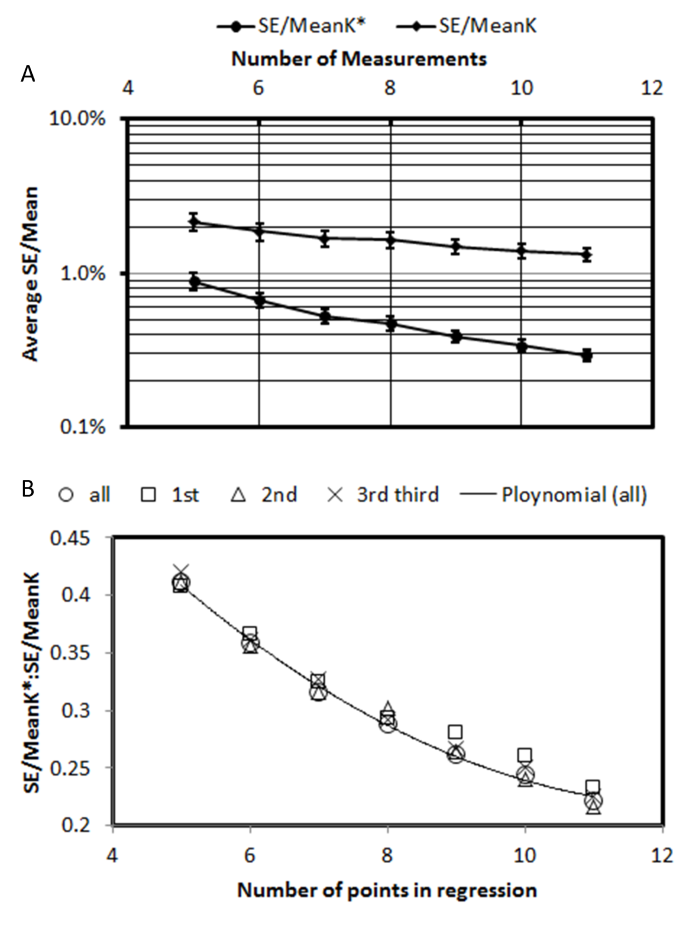Figure 3. This shows a comparison of the error relative to the mean (SE/mean) of the regression and traditional methods. Panel A: relative SE/mean versus number of measurements, the diamond refers to the average value SE/mean computed from all the points in the VC in Fig1A.The circles represent the average SE/mean by regression method (averaged over the entire VC). Panel B: the ratio of two relative errors versus the number of measurements. Open circles represent the ratio between regression and tradition methods of SE/Means from the whole curve; open squares, open triangles and cross represent the ratio of two means at the first, second and third part of the vulnerability curve respectively. The smooth line is a polynomial fit for the open circle values.

Average SE/ (traditional method) and SE/* (regression method) were plotted in Figure 3A, we can see that average SE/*s were always lower than SE/ and that standard errors of SE/*s were always lower than those of SE/K. Figure 3A showed that with the increase of N, both methods resulted in better precision and lower deviation of SE/Mean, but regression approach yielded an average SE/Mean which converged to lower values faster than the traditional method as proven by the ratio in Figure 3B. Significantly, when we collected ≥ 11 points for regression, the error was less than 0.3%, moreover, the time required to collect 11 points was < 30 s  more than collecting 5 points.

### Temperature regulation

Without refrigeration, the air temperature inside a centrifuge experienced by the rotor will increase with rotor speed because of the heat dissapation of the rotor motor. The proper physical design of the heating-cooling systems of centrifuges is essential to achieve accurate temperature control. Critial design fators include the type of refrigeraton system used and the location of the thermostatic temperature sensor. Temperature control by refrigeration is better if the system can both heat and cool (a reversible heat pump). If the refrigeration system can only be turned on and off (standard refrigeration) then the responsiveness of the sytem is compromised in the heating phase because heat is derived only by passive heat flow from the rotor motor and from the ambient lab temperature. The placement of the thermostatic temperature sensor is also critical to good temperature control, because if the sensor used by the thermostat is placed remotely from the rotor then the rotor could be at quite a different temperature than the sensor location. Improper location of the thermostat-sensor will degrade the quality of temperature control even if refrigeration control is of the highest quality.

We have recently taken delivery of a new cavitron system from Xiang Yi Instrument Co. (a modified model H2100R centrifuge), which was capable of controlling and setting air temperature to the nearest 0.1 oC. The heat pump in the Xiang Yi centrifuge has a ‘reversing valve’, hence the heat pump actively heats and cools whereas the Beckmann-Coulter Centrifuge (Allegra X-22R) only cools making the air temperature fluctuate by ±2 oC when the thermostatic setting is unchanged at constant RPM (see Fig. 4A). Also there was often a large temperature gradient in the Beckmann-Coulter centrifuge between the temperature sensor of the centrifuge and temperature independently measured near the rotor, and the temperature gradient increased with RPM (see Fig. 4B). We compensated for this temperature gradient by progressively decreasing the thermostat setting as RPM increases according to the actual air temperature measured with our own temperature sensor placed near the rotor (see Fig. 4C). The temperature gradient within the Xiang Yi is negligible because of the better placement of the thermostat temperature sensor. Hence the accurate control of temperature and viscosity is solved by the more precise temperature control of the Xiang Yi cavitron.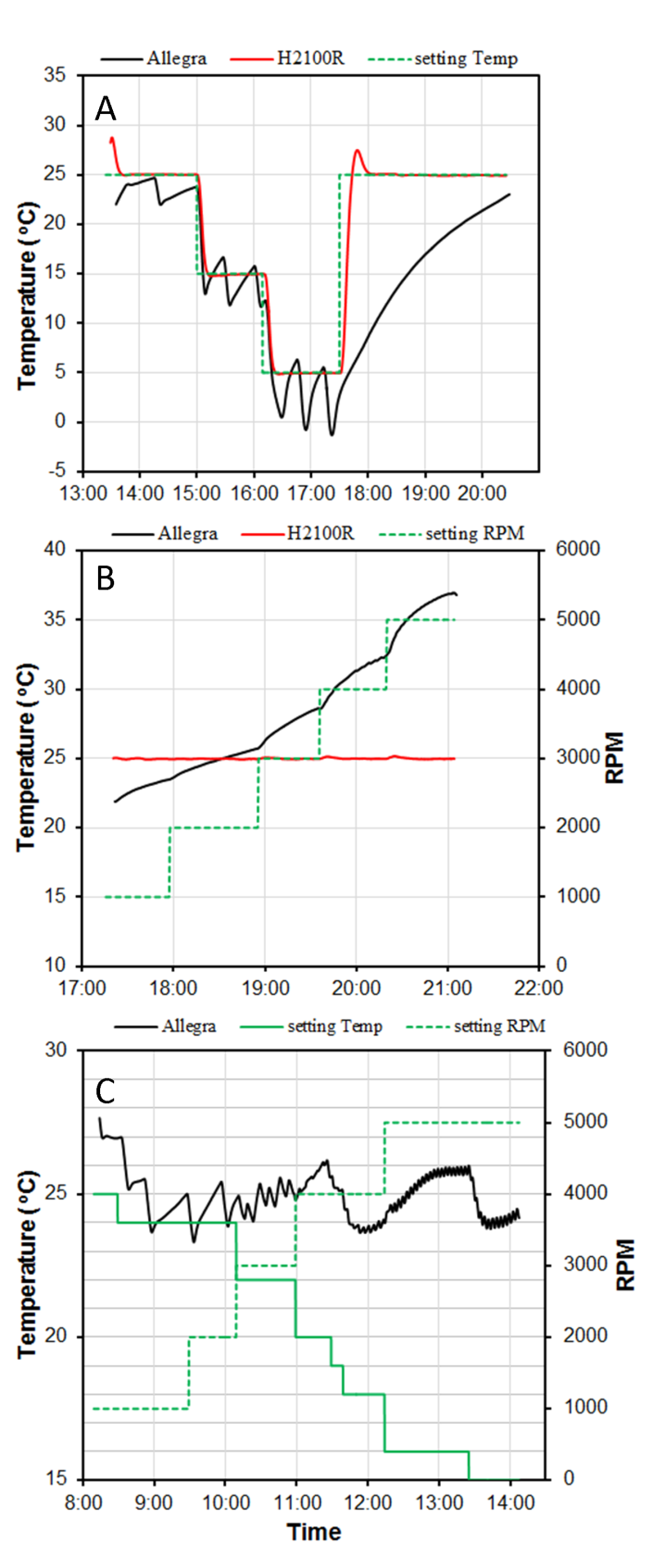Figure 4. This figure compares the ability of two centrifuges to control the air temperature near the rotor. Allegra = the Beckman-Coulter model Allegra X-22R and H2100R = the XiangYi model H2100R. A. This shows the air temperature near the rotor while spinning at a constant 1000 RPM but changing the temperature set-point of the centrifuge. B. Air temperature near the rotor while keeping the temperature set point at 25 oC while increasing RPM. C. Air temperature near the rotor in the Allegra while adjusting the temperature setting to compensate for heating due to RPM increase.

How Tstem depends on the air temperature.

Because the value of Tstem cannot be measured directly, the uncertainty of the stem temperature had a large impact on values of K, hence we performed an experiment to find an algorithm to compute Tstem even when Tair inside the centrifuge changes dynamically with time. The experiment is illustrated in Fig. 5.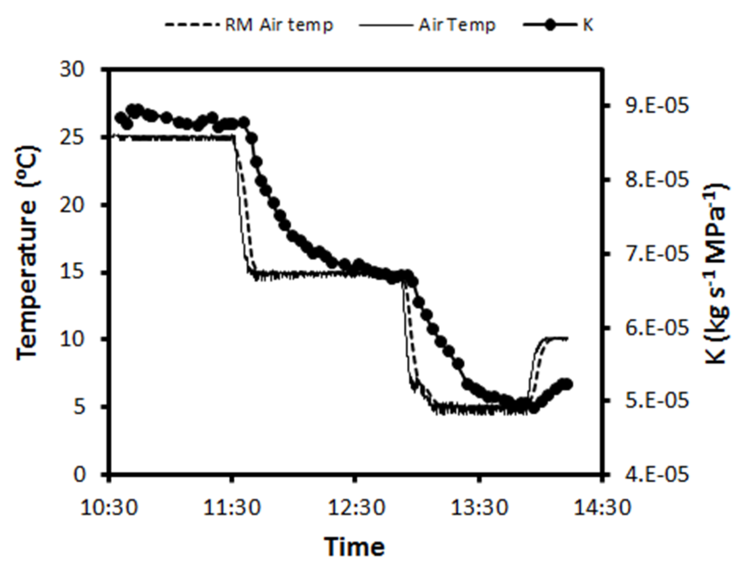Figure 5 shows the tempo of change in centrifuge air temperature, 5-min running mean air temperature and K measured on a Populus 84K clone. The solid line is the experimentally imposed change in air temperature. The solid circles show the response of the stem temperature.

The XiangYi Centrifuge was adjusted to three different constant temperature values of 25, 15 and 5 oC. The thermostat of the centrifuge could maintain a constant air temperature with a SD of ±0.11, 0.17 and 0.29 oC at the thermostat settings of 25, 15 and 5 oC, respectively. The 5 min running mean of that air temperature was a smooth line with small SE < ± 0.04 oC at all set-points. The stem conductance, K, was measured repeatedly and declined slowly compared to the air-temperature values indicating that the Tstem declined much more slowly than the air temperature. The tempo of decline of K is primarily a function of Tstem, but it is not exactly exponential because the last half of the tempo has a half time of about 17 min but the first half was closer to 10 min.

Fig. 6 shows the correlation between several running mean air temperatures and K. The best correlation was obtained with a running mean air temperature between 30 and 35 minutes. But a much stronger correlation was found using the first order rate model (Eq. 6, see methods) and a running mean value of 5 min for the air temperature. However the correlation was nearly as good using the actual air temperature read at 5 s intervals (data not shown). The combined model (Fig. 5D) worked best because the stem was deep inside the rotor and there would be a small time delay (a few minutes) between when the temperature changed in the air of the centrifuge and when the temperature changed in the metal next to the stem. The 5 min running mean air temperature seemed to simulate this time delay adequately. The curve in Fig. 5D was fitted using the solver function in Excel which adjusted two parameters to obtain a best fit. The parameters were α and the initial Tstem in Eq. (6). The best fit values were α = 9.942E-4 s-1 and Tstem,0 = 25.74 oC.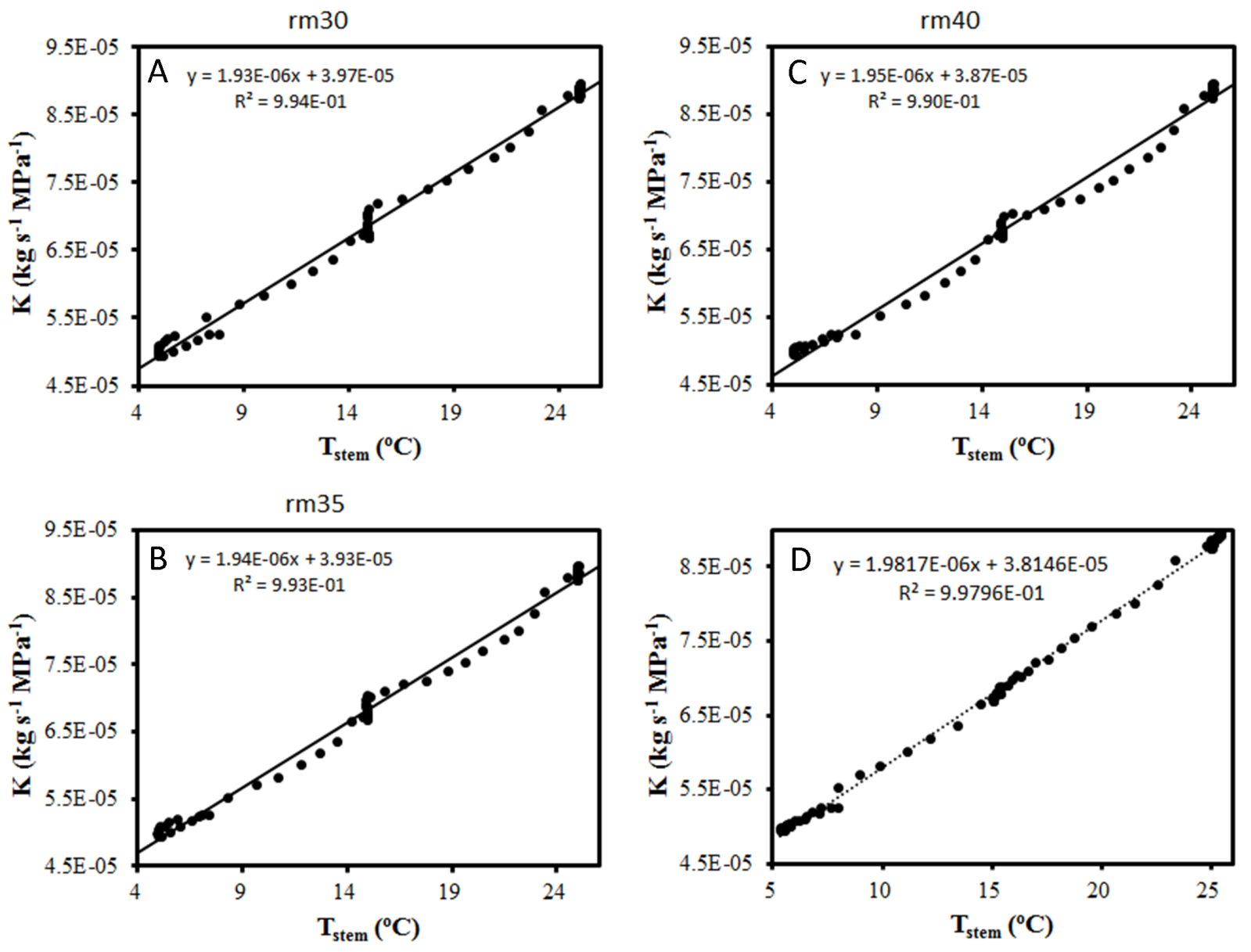Figure 6 shows how models of Tstem correlate with K. The three best running mean models (Eq. 5, see methods) are shown in A, B, and C for running means of 25, 30 and 35 min, respectively. Graph D shows the fit for Eq. 6 (see methods), which was a first-order rate reaction model using a 5 min running mean air temperature as the input.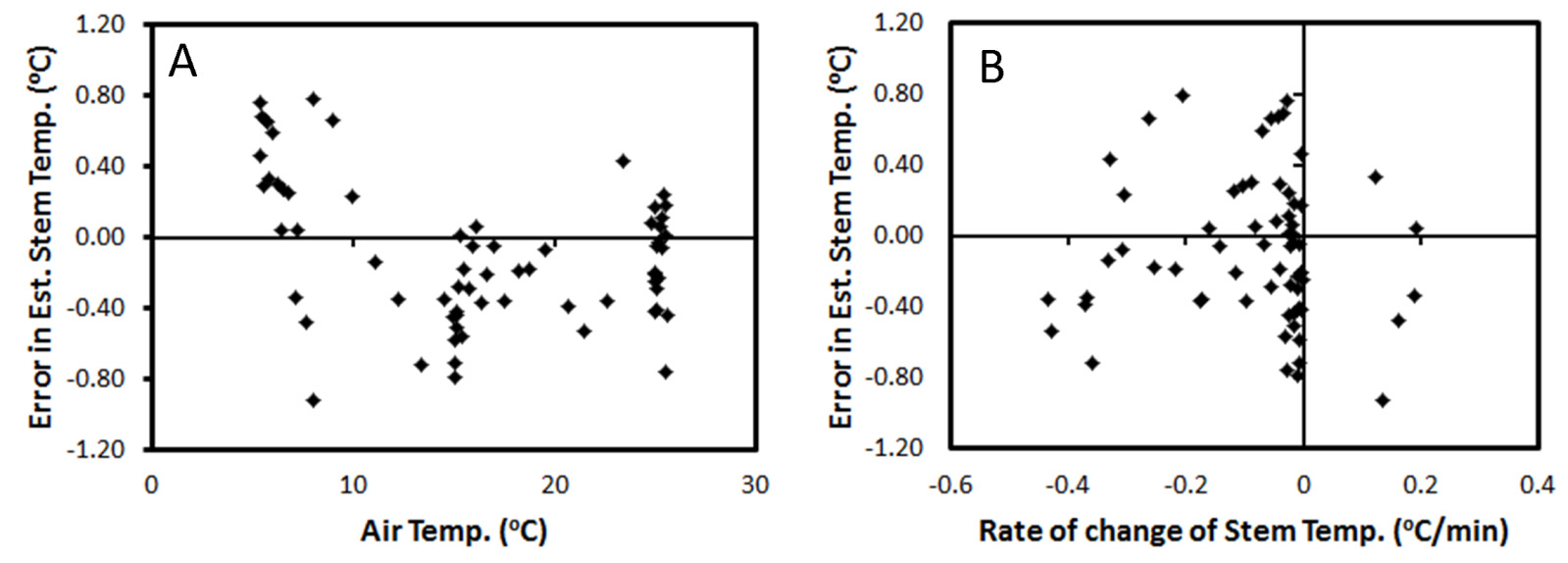Figure 7. This shows our estimate of the error in estimation of Tstem computed from the residuals in Fig. 5D. See text for details. A: is the temperature error as a function of Tstem and D: is the temperature error as a function of the speed of temperature change in the original experiment (Fig. 4). The Tstem is probably more stable than indicated in by the y-axis values because some of the error can be accounted for by the error in measuring K whereas the error values shown assume all errors are due to changes in 1/η.

It was surprising to us that Tstem could be fitted to a first order thermal rate reaction theory, but it is common practice to ‘lump’ temperature changes in this way when the thermal diffusivity of the substances surrounding an object is > the thermal diffusivity of the object. Thermal diffusivity is defined as D=h/ρCp, here h is the thermal heat transfer coefficient, ρ is the density of the material and Cp is the heat capacity. When D is used to compute thermal equilibration, equations analogous to Fick’s law can be used to compute changes in T in place of concentration. The values of D (m2 s-1) for the aluminum rotor, air and stem were ~6X10-5, 1.9x0-5 and 0.014x10-5, respectively, (assuming stem D = the value of water). Hence the condition for the first order thermal rate reaction approximation was met.

In order to achieve a K-measurement accuracy of ±0.3% we need to prove that temperature changes less than ±0.1 oC over the period of measurement of one K-value. However, if we want to measure a highly-accurate vulnerability curve then we need to compute many values of percent loss of hydraulic conductivity, PLC = 100%(1-K/Kmax); in this case the temperature has to be constant to ±0.1 oC for Kmax as well as all other K values measured over a period of 1 h or more. The residuals in Fig. 7 prove that if the air temperature inside the centrifuge changes dynamically the Tstem is known to only ±0.4 oC which results in an error of PLC of about ±1%. The control of Tstem is better if air temperature does not change dynamically.

If highly accurate vulnerability curves are desired, then the temperature of the stem must to controlled to <±0.1 oC. If a Cochard rotor is used then this highly accurate temperature control has to be maintained inside the centrifuge that spins the rotor. If a Sperry rotor is used then the temperature has to be controlled outside the centrifuge because K is measured in a conductivity apparatus. Since an air-conditioned room-temperature usually fluctuates ± 1 or 2 oC, we would recommend keeping stems immersed in a constant temperature bath accurate to ±0.05 oC and waiting for thermal equilibrium between the bath and stem before commencing the determination of K. If a Cochard rotor is used then all K-measurements are made while the rotor is spinning. In this case the selection of a centrifuge with good air-temperature control is essential.

As illustrated in Fig. 4 the temperature regulation of the H2100R is superior to the Allegra X-22R. The Allegra X-22R has a 5-min running mean air temperature fluctuation of ±2 oC, as measured with an independent LM335 temperature sensor mounted near the rotor. In addition a thermal gradient between the rotor and internal temperature sensor, used by the Allegra X-22R to control the refrigeration, caused large deviations in mean air temperature as rotor RPM increased due to the heat generated by the rotor-motor. In contrast, the H2100R controlled the 5-min running mean air-temperature within ±0.04 oC (except when there is a large step-increase in the thermostat setting), hence we are confident that Tstem can be stabilized to within <±0.1 oC. To achieve this level of temperature stability we recommend setting the H2100R equal to the mean lab air temperature and spinning the stem for at least 1 h prior to measuring the vulnerability curve to be sure that the Tstem has approached a stable temperature. If there is reason to believe the initial Tstem differed from the mean lab temperature by more than 1 oC prior to placement in the centrifuge then a 1.5 to 2 h equilibration period might be warranted prior to commencing the measurement of a vulnerability curve.

Having addressed the two main sources of error in the computation of K and PLC, we need to briefly review how other factor lead to a propagation of errors. For PLC the situation is simple because PLC/100 = 1-K/Kmax =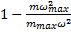. Here we can use the general rule for propogation of errors: if A = BC/D and the error of each parameter is ±a, ±b etc then the estimated relative error of A is given by:In the case of PLC computation the slopes m and mmax have the biggest errors whereas in modern centrifuges the anglular velocity, ω, has a relative error <0.04% for most of the vulnerability curve. Since the error of m and mmax is about an order of magnitude more and equal, the overall error on PLC ≅ √2 x (the error of m or mmax).

In contrast if the objective is to measure K in a cavitron rotor then the errors to be considered are based on the math of Eq. (2) and for Kh (conductivity = K*L) there is the uncertainty of L to be added. We leave it to the reader to estimate the likely uncertainty of Aw, R, and L, but we would estimate the combined uncertainty to be about 1.2% or more.

In conclusion, the method we developed increased the precision of hydraulic conductance by a factor of 4~5 times, resulting in an improved precision as measured by SE/mean when a regression was done on 10 to 12 points which usually required less than 1 min per regression. Further improvements in the absolute precision of K measurement below ±1% would require improved methods of measuring and/or cutting L, R, and stem diameter. Paying attention to temperature stability and precision of measurement of K will improve the precision of vulnerability curves. In our opinion minor deviations in the shape of vulnerability curves may be correlated with xylem anatomy in the future so more recise mesaurement techiniques are needed. We recommend the methods used in this paper to anyone using a Cochard cavitron to measure vulnerability cuves.

Acknowledgements : this research was made possible by a 5-year 1000-talents research grant awarded to MTT.

# Authors

#### Yujie Wang

Affiliation : College of Forestry, Northwest A&F University, Yangling, Shaanxi 712100, China

#### Régis Burlett

Affiliation : INRA, University of Bordeaux, UMR BIOGECO, 33405 Talence, France

#### Feng Feng

Affiliation : College of Forestry, Northwest A&F University, Yangling, Shaanxi 712100, China

#### Melvin T. Tyree

Affiliation : College of Forestry, Northwest A&F University, Yangling, Shaanxi 712100, China

# Attachments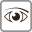Views: 761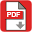PDF: 140